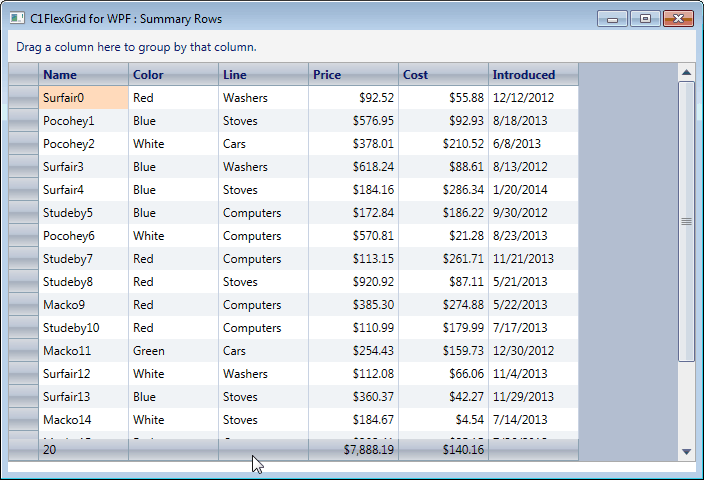﻿ Summary Row in C1FlexGrid# Summary Row in C1FlexGrid

When using a Grid control, many of us have a requirement of displaying some kind of information on the Footer Rows or the Summary Rows of the grid. In this blog post, we will discuss how a summary row can be added in ComponentOne FlexGrid for WPF/Silverlight.

## Let's take a quick look at the approach being used

It is basically a Footer Row which will be added to the ColumnFooters.Rows Collection of the C1FlexGrid. If you wish to display the same information on the Topmost row of the grid instead of at the end, you can do that as well. Just add the respective row in the ColumnHeaders.Rows collection of the grid ;)

## Implementation

The implementation involves the following steps : 1. Display some information in a Grid, for instance , Name, Color, Line, Price, Cost and introduction of some products in a Company. 2. Display the total number of products, average of their costs and sum their prices in the SummaryRow placed at the end of the Grid.

## Binding the Grid

1. Assign an ItemSource to the C1FlexGrid.

``````
// create a data source
var list = new List<Product>();
for (int i = 0; i < 20; i++)
{
}
// assign the data source to both grids
var cvs = new CollectionViewSource() { Source = list };
_flex.ItemsSource = cvs.View;

``````

2. The Xaml Definition looks like:

``````
<c1:C1FlexGrid x:Name="_flex" Grid.Row="1" AutoGenerateColumns="False">
<c1:C1FlexGrid.Columns>
<c1:Column Binding="{Binding Name,Mode=OneWay}"/>
<c1:Column Binding="{Binding Color, Mode=TwoWay}"/>
<c1:Column Binding="{Binding Line}"/>
<c1:Column Binding="{Binding Price, Mode=TwoWay}" Format="N2"/>
<c1:Column Binding="{Binding Cost, Mode=TwoWay}" Format="N2"/>
<c1:Column Binding="{Binding Introduced}" Format="d"/>
</c1:C1FlexGrid.Columns>
</c1:C1FlexGrid>

``````

So, to add a footer row, all you have to create a group row and add that as the ColumnFooter row.

``````
var gr = new C1.WPF.FlexGrid.GroupRow();

``````

The final Xaml MarkUp looks like:

``````
<c1:C1FlexGrid x:Name="_flex" Grid.Row="1" AutoGenerateColumns="False">
<c1:C1FlexGrid.Columns>
<c1:Column Binding="{Binding Name,Mode=OneWay}" GroupAggregate="Count"/>
<c1:Column Binding="{Binding Color, Mode=TwoWay}"/>
<c1:Column Binding="{Binding Line}"/>
<c1:Column Binding="{Binding Price, Mode=TwoWay}" Format="N2" GroupAggregate="Sum"/>
<c1:Column Binding="{Binding Cost, Mode=TwoWay}" Format="N2" GroupAggregate="Average"/>
<c1:Column Binding="{Binding Introduced}" Format="d"/>
</c1:C1FlexGrid.Columns>
</c1:C1FlexGrid>

``````

Here is the final output.Therefore, we have the following data: 1. Number of Products- 20 2. Sum of their Prices- \$7,888.19 3. Average of their Costs- \$140.16 Download SL Sample Download WPF SampleGrapeCity

GrapeCity Developer Tools